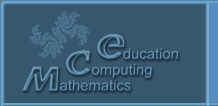Русский
 ! Вопросы и ответы

# Abstracts

## The ray algebras and space-time simmetry

Koganov A.V.

Federal Science Center System Research Institute of Russian Academy of Sciences (FGU FNC NIISI RAN) (SRISA) Russia, Moscow, 117218, Nakhimovsky st., 36, corpus 1, koganow@niisi.msk.ru

1 pp. (accepted)

The symmetries of multidimensional linear spaces are often excessive with respect to the symmetries of the simulated objects (for example, the time arrow violates the symmetry of the numerical line). We construct a class of numerical algebras that have a restriction of degrees of freedom, similar to the violation of the symmetry of time. These algebras and the corresponding numbers are proposed to be called ray. In them, negative numbers are considered as a complex extension of the algebra of non-negative numbers, (-1) becomes a new complex unit, and each complex unit of Hypercomplex algebras is equipped with a dual negative complex unit. The base algebra becomes a nonnegative half-axis (ray) with operations of addition (semigroup) and multiplication (group and ideal 0). In these algebras there are no zero divisors, but division is possible not for any pairs of numbers, but only if there are nonnegative solutions of some system of linear equations. All ray algebras are modular on both operations for the norm L1. Their dimensions are twice the dimensions of the corresponding Hypercomplex algebras (analogues), but they cannot be considered as an extension of these algebras, although there are automorphisms of ray algebras on analogues. Inverse automorphisms of a standard requires some modification of radiation operations. An example of efficiency: these algebras make it possible to strengthen the Wheeler-Feynman postulate and obtain in the theory of relativity a proof of the impossibility of transmitting a nonzero action outside the light cone and back in time in the light cone. With complex algebra, it was previously possible to prove only the first part of this statement. The work was done on the topic of the state task of research 0065-2018-0004.

© 2004 Designed by Lyceum of Informational Technologies №1533